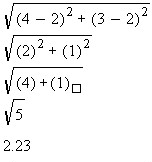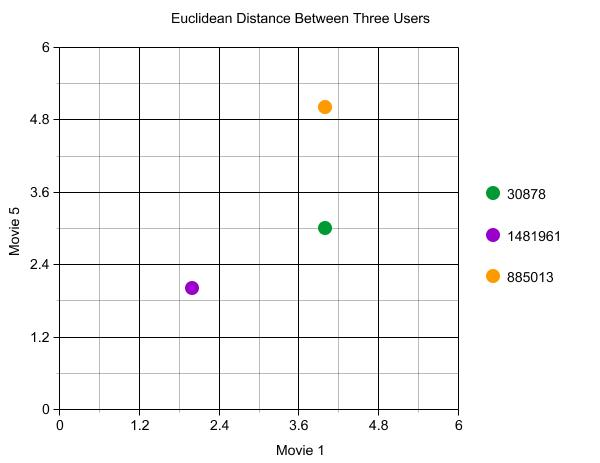# Comparing Distance Formulas. Part III

This article will cover the Euclidean Formula. I will cover the basic equation for the algorithm, provide running and working examples for both Ruby and PHP5, but will state advantages and disadvantageous of the algorithm after
covering every algorithm in the article.

Background – Euclidean Algorithm
One of the oldest and one of the most widely used distance formulas is the Euclidean formula. The formula captures two items and compares each attribute of each item to one another to determine how close, related, they
are. In other words. With the formula we can take two users run through each of their characteristics, in our case movie ratings, and determine how similar they are.

But how is the value that the algorithm provides us used? Once the calculations are done between two users we are presented with a value between 0 and infinity. The larger the value the farther apart both users are to one another (not similar). A few example of what values may be provided are 0.56, 2.89, 4. These are the values that will be used to determine how similar both users are to each other.

The Math EquationThe equation above gives us both the short hand and the expanded version. We’ll take a look at the short hand. In short hand the equation takes the difference between the attributes of two objects specified in the (Pi-Q1) portion and squares it. For a single dimension, attribute, the equation will take the square-root and then finish. Fortunately for us, the equation allows us to use more than one attribute so it continues by taking the sum of the next set of attribute differences until there are no additional attributes to compare.

Calculating Distances with an example.
Using the notation above, lets take a simple example using our data set to make it clear what the equation is doing. For this example well use 3 users and 2 movies all users rated.

1:
30878,4,2005-12-26
1481961,2,2005-05-24
885013,4,2005-10-19

5:
30878,3,2004-10-19
1481961,2,2005-09-27
885013,5,2005-05-15

The data sets we are using for this example has three users, user with id 30878, id 1481961, and id 885013 rating two movies. One movie with id 1 and the other with id 5. User 30878 gave the movie 1 a rating of 4 and gave movie 5 a rating of 3. User 1481961 gave movie 1 a rating of 2 and gave a rating of 2 to movie 5. User 885013 gave movie id 1 a rating of 4 and a rating of 5 to movie id 5.

Preparing the above data to use with the Euclidean formula, we convert each user to either a P or Q. The goal is to determine to distance between user 30878 and both 1481961 and 885013. 30878 will be represented by the letter P and Q will be the user 1481961 for the first distance calculation. The second distance calculations will simply change the Q to represent the user 885013. Also, since there are only two movies, dimensions, we know that the equation will take the calculation for 2 dimensions.

Distance between user 30878 and 1481961Distance between user 30878 and 885013

After calculating the distance between both users what does the final numerical value represent? It simply tells us that the distance, or the similarity between both users is 2.23. The rule of thumb is, as the results decrease in value the similarity between each user increases. If the result was a 3.5 or 2.89 the similarity between the users would decrease while a result of 0 or 0.2 would indicate an extremely similar user.

Visualizing Similarity with a Graph
Yet another way to view the data is graphically. Plotting the users on a X/Y graph where the Y-axis represents one dimension, ratings for video 5, and the X-axis represents ratings for video 1 we can visually see how close the users are to one another.User 30878 has the coordinates, (4,3), while user 1481961 has coordinates (2,2), and user has coordinates (4,5). The graph describes how close, similar, each user is to the user 30878. User 1481961 is closer to 30878 compared to 885013, thereby making these two users similar.

The Code

PHP5 Class

```/** * Distance Formula Class * * @author Armando Padilla, mando81@prodigy.net * @copyright Armando Padilla. * */ class DistanceFormulas {```

public function __construct(){}

public function euclidean(array \$objectXAttribute, array \$objectYAttribute){

\$distanceFromXToY = 0;
for(\$i=0; \$i<count(\$objectXAttribute); \$i++){

\$p = \$objectXAttribute[\$i];
\$q = \$objectYAttribute[\$i];

\$distanceFromXToY += pow((\$p-\$q), 2);

}

return \$distanceFromXToY;

}//End

}//End class

PHP5 Test

/**
* Simple example using the DistanceFormula Class
*
*/
require_once(“DistanceFormulas.php”);

//List of users along with their ratings.
//usually you would have this in a database and have more than 3 users 🙂
//
// rating order: “The Abyss”, “Hunt for Red October”, “Goonies”
\$profiles = array(array(“username” => ‘Armando’, “movieratings” => array(5, 4, 4)),
array(“username” => ‘Snoopy’, “movieratings” => array(2,2,5)),
array(“username” => ‘Pearl’, “movieratings” => array(1,4,1)));

//Instantiate the class
\$DistanceFormula = new DistanceFormulas();

//Let’s get the distance from Armando to the other two users.
for(\$i=1; \$i<count(\$profiles); \$i++){